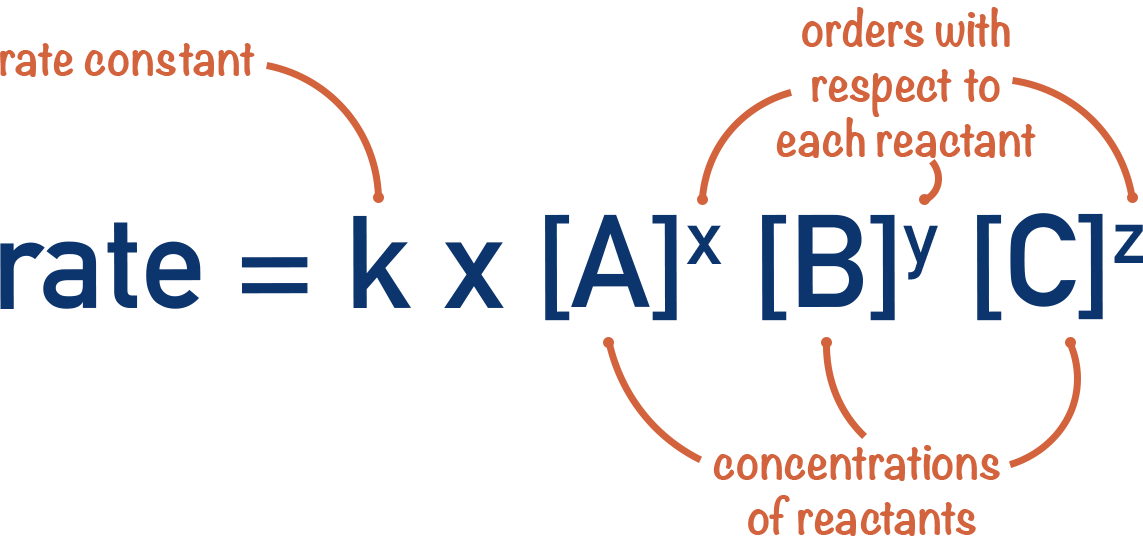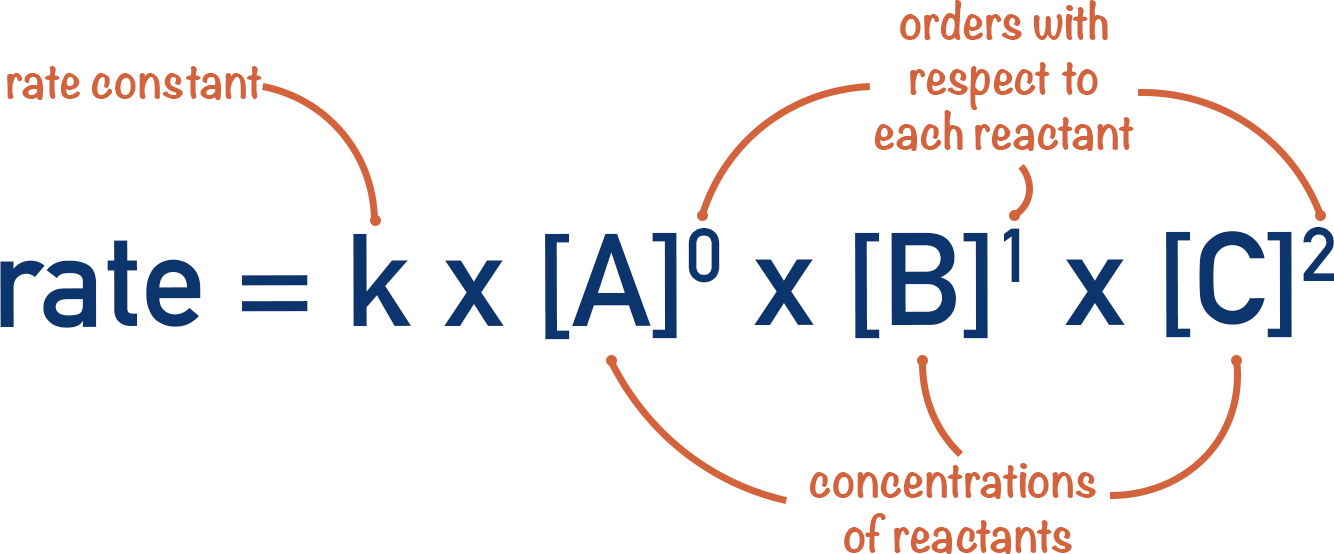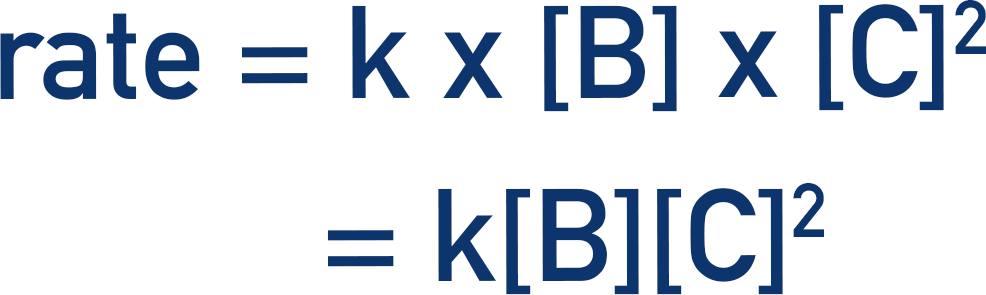Quick Notes The Rate Equation

• A rate equation links the rate of a reaction at a particular temperature to the concentrations of all reactants.
• A rate constant, k, is used in the rate equation to account for the influence of temperature.
• A rate constant is only for a given temperature.
• Different reactions can have different rate constant values and the units can also be different.
• In the rate equation, each concentration of reactant is raised to the power of its order and multiplied by the rate constant, k.Full Notes The Rate Equation

If we know all of the orders with respect to reactants for a reaction, we can link them all together to see how the rate of a reaction changes when we change their concentrations. To do this, we use a rate equation.

It is not just the concentrations of reactants that influences the rate of a reaction. The rate of a reaction also changes with temperature and a small change in temperature can lead to a big change in a rate of reaction. A rate constant, k, is used to link the effect of concentrations at a particular temperature to the rate of a reaction.

Key point: a rate constant, k, is only for a given temperature. If you change the temperature the reaction is being carried out at, the value of k also changes. Every reaction has a different value for k and the units are not fixed (meaning k can have different units for different reactions).If we wanted to construct a rate equation for the above example, we have to combine the individual orders for all the reactants and multiply them by the rate constant, k.

For example, if reaction is zero order with respect to A, first order with respect to B and second order with respect to C.Each concentration of reactant is raised to the power of its order.Anything raised to the power zero equals one, therefore [A]0 = 1. This means we can now simply put one in the rate equation instead of [A]0Anything raised to the power one just equals itself, [B]1 = [B].We now have a rate equation that shows how changing the concentration of a particular reactant will change the rate of reaction, at a particular temperature.